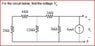# Circuit Analysis: Find V_0 for R_eq = 3kOhm and I_s = 6mA

• Engineering
• Firestrider

## Homework Statement

Code:
|------|----a
|      |^   +
|      ||
R_eq   I_s  V_0
|      |
|      |    -
|------|----b

R_eq = 3kOhm
I_s = 6mA

Find V_0.

## Homework Equations

Ohm's law: KVL, KCL

## The Attempt at a Solution

Should this work just like any other circuit where V = IR? So V = (6 x 10^-3)(3 x 10^3) = 18? Or does it work differently since V_0 is an open circuit.

Here is the original problem:

#### Attachments

•CAp2.JPG
12.7 KB · Views: 391

It should work like any other circuit. This problem is asking for the difference in potential between the top node and bottom node of the circuit, which is labeled as V0. However, you are getting the wrong answer because your Req is off. Try recombining the resistors again and you should get it.Ok well the first two circuits on the left can connect in series to make 6kohm, then the circuits can connect in parallel to make 4kohm, then connect in series to make 6kohm, and then in parallel again to make 2kohm.

So, V_0 = 6 x 10^-3 * 2 x 10^3 = 12V?

I have 2 other problems attached. I'm not sure how to go about them. I know KVL, KCL, and Ohm's law.

I also know if resistors are in series I can do a voltage divider and if resistors are in parallel I can do a current divider.

#### Attachments

Last edited:
Yes, your solution for the first one is correct.

If you make an attempt on the other 2 problems and post some work, I can try to help you once the images are approved.